In the circuit shown the cells A and B have negligible resistances. For  the galvanometer (g) shown no deflection.The value of ${V}_{B}$ is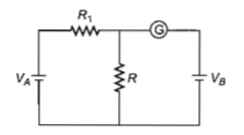(a)4V                                         (b)2V

(c)12V                                       (d)6V

High Yielding Test Series + Question Bank - NEET 2020

Difficulty Level:

If voltage across a bulb rated 220 V-100 W drops by 2.5% of its rated value, the percentage of the rated value by which the power would decrease is

(a)20%                                             (b)2.5%

(c)5%                                               (d)10%

High Yielding Test Series + Question Bank - NEET 2020

Difficulty Level:

A ring is made of a wire having a resistane

${\mathrm{R}}_{0}=12\mathrm{\Omega }$. Find the points A and B, as shown

in the figure, at which a current carrying

conductor should be connected so that the

resistance R of the sub circuit between these

points is equal to 8/3$\mathrm{\Omega }$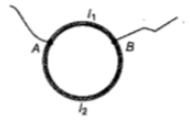1. $\frac{{\mathrm{l}}_{1}}{{\mathrm{l}}_{2}}=\frac{5}{8}$                    2. $\frac{{\mathrm{l}}_{1}}{{\mathrm{l}}_{2}}=\frac{1}{3}$

3. $\frac{{\mathrm{l}}_{1}}{{\mathrm{l}}_{2}}=\frac{3}{8}$                    4. $\frac{{\mathrm{l}}_{1}}{{\mathrm{l}}_{2}}=\frac{1}{2}$

Concept Questions :-

Combination of resistors
High Yielding Test Series + Question Bank - NEET 2020

Difficulty Level:

The power dissipated in the circuit shown in

the figure is 30 Watt. The value of R is :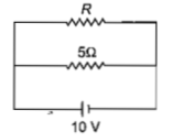(a) $20\mathrm{\Omega }$

(b) $15\mathrm{\Omega }$

(c) $10\mathrm{\Omega }$

(d) $30\mathrm{\Omega }$

Concept Questions :-

Heating effect of current
High Yielding Test Series + Question Bank - NEET 2020

Difficulty Level:

A cell having an emf $\mathrm{\epsilon }$ internal resistance r

is connected across a variable external

resistance R. As the resistance R is increased,

the plot of potential difference V across R is given

by

(a)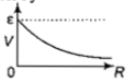(b)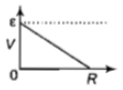(c)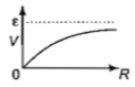(d)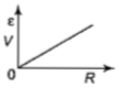Concept Questions :-

Emf and terminal voltage
High Yielding Test Series + Question Bank - NEET 2020

Difficulty Level:

If power dissipated in the 9$\mathrm{\Omega }$ resistor in the circuit

shown is 36 W, the potential difference across

the 2$\mathrm{\Omega }$ resistor is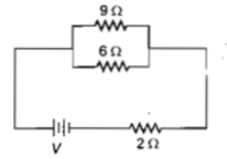(a) 8 V

(b) 10 V

(c) 2 V

(d) 4 V

Concept Questions :-

Heating effect of current
High Yielding Test Series + Question Bank - NEET 2020

Difficulty Level:

A current of 2A flows through a 2$\mathrm{\Omega }$ resistor

when connected across a battery. The same

battery supplies a current of 0.5 A when

connected across a 9$\mathrm{\Omega }$ resistor. The internal

resistance of the battery is

(a) $1/3\mathrm{\Omega }$                   (b) $1/4\mathrm{\Omega }$

(c) $1\mathrm{\Omega }$                       (d) $0.5\mathrm{\Omega }$

Concept Questions :-

Emf and terminal voltage
High Yielding Test Series + Question Bank - NEET 2020

Difficulty Level:

In the circuit in the figure, if the potential at point A is taken to be zero, the potential at point B is :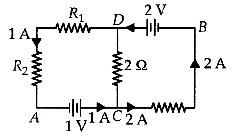1. -1V                                          2. +2V

3. -2V                                          4. +1V

Concept Questions :-

Kirchoff's voltage law
High Yielding Test Series + Question Bank - NEET 2020

Difficulty Level:

Which one of the following bonds produces a solid that reflects light in the visible region and whose electrical conductivity decreases with temperature and has high melting point?

(a) metallic bonding

(b) van der Waals'bonding

(c) ionic bonding

(d) covalent bonding

Concept Questions :-

Current and current density
High Yielding Test Series + Question Bank - NEET 2020

Difficulty Level:

A potentiometer circuit is set up as shown.The potential gradient across the potentiometer wire, is k volt/cm and the ammeter, present in the circuit, reads 1.0A when two way key is switched off. The balance points, when the key between the terminals (i) 1 and 2 (ii) 1and 3, is plugged in, are found to be at lengths  respectively.The magnitudes, of the resistors R and X, in ohm, are then, equal, respectively, to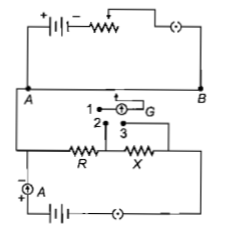(a)

(b)

(c)

(d)

Concept Questions :-

Meter bridge and potentiometer